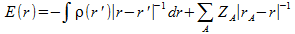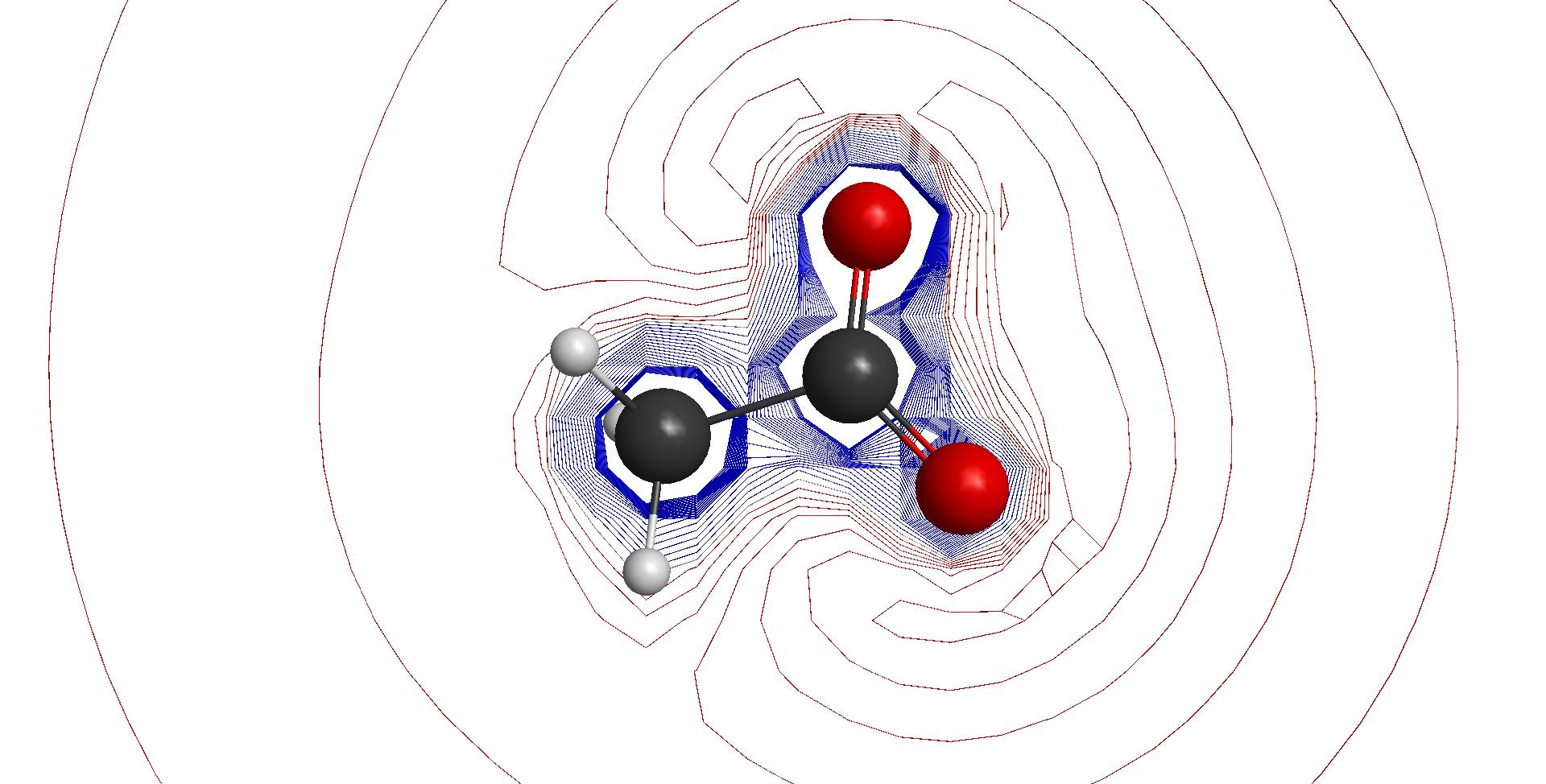# Quantumbiochemistry.org

## Some Basic Principles:

A chemical reaction is a clearly observable change in a substance that is typical for that substance.

When a substance undergoes a chemical reaction then there will be a rearrangement of the nuclei and the electrons. The rearrangements that are possible are typical for the substance. Because of this there is a theory that relates the chemical structure to the possible chemical reactions.

## The role of lewis acid and base reactions in biology:

A Lewis acid is a molecule that can accept an electron pair from another molecule the Lewis base. Most chemical reactions in biology are Lewis acid-base reactions. Biologists, biochemists, bioengineers need to understand the reactions between metabolites.

In biological organisms metabolites react often in the presence of macromolecules (like polypeptides, carbohydrates, lipids, DNA, ... ) water and minerals. These substances act as chemical catalysis or as inhibitors.

Biological organisms manage their chemical reactions by means of catalysis and inhibition. Through evolution they developed catalytic mechanisms that change the reaction mechanism of a Lewis acid and base reaction. They control events even at the subatomic level. This way they can control when, where and how fast the reaction occurs and what products are formed. The information on how to do that is encoded on DNA the molecular carrier of genetic material.

The Lewis acid-base theory will enable us to understand the way macromolecules, water and ions interact.

## Quantum chemical principles on Lewis acid-base reactions :

Quantum chemistry allows us to make a comprehensive theoretical analysis on the mechanisms that govern a Lewis acid-base reaction.

The rate at which the reaction occurs and the nature of the products depends on the reaction mechanism.

There are essentially two ways a Lewis acid reacts with a Lewis base, the reaction can be charge controlled or it can be frontier controlled. These principles will be important at the first onset of a reaction. At a later phase new bonds appear and existing bonds disappear and a rearrangement of the nuclear positions can occur. One or more reaction intermediates can exist in small quantities and the properties of these intermediates further determine the course of the reaction.

When a reaction is charge controlledthe reaction will depend mostly on the coulomb charge due the electron cloud of the reacting molecules. When the energy gap between the highest occupied molecular orbital and the lowest unoccupied molecular orbital is large the reaction will be charge controlled. In this case the molecular electrostatic potential will provide information on how the Lewis acid can accept an electron from the Lewis base. The molecular electrostatic potential at point p(x,y,z) is the electrostatic force acting on a positive point charge located at this point p through the electron charge cloud.

A frontier controlled reaction will depend on the overlap between the lowest unoccupied molecular orbital (LUMO) of the Lewis acid and the highest occupied molecular orbital (HOMO) of the Lewis base. When the energy gap between the LUMO and the HOMO is small a reaction will be frontier controlled. The shape of the LUMO and the HOMO determine the possibilities for overlap and thus determines the initial course of the reaction.

Kenichi Fukui originally proposed the frontier molecular orbital theory. See G. Klopman, Chemical Reactivity and Reactions Paths , p23-50 Wiley, New York, 1974. Fukui modeled a charge transfer by considering 3 situations.

• Lewis Acid A and Lewis Base B are both in their ground state, no charge transfer occurs.
• Lewis base B has one electron excited, Lewis acid A is in its ground state.
• Lewis base B has transferred one electron to Lewis acid A.

Each situation can be represented by a Slater determinant and a Hamiltonian can be defined for that system. As a consequence one can apply the variational principle to the model. By analysing the resulting equation he could explain the principle of charge controlled and frontier controlled reactions.

Independently Gilles Klopman proposed the generalised perturbation theory of chemical reactivity. This theory explains the principles about Lewis acid-base reactions outlined here. A comprehensive text on this subject can be found in G. Klopman, Chemical Reactivity and Reactions Paths , p55 Wiley, New York, 1974.

Raplh G. Pearson first explained the Hard and Soft Acis and Bases Principle. See Ralph G. Pearson "Hard and Soft Acids and Bases" Journal of the american chemical society Vol 85, 22 November 20, 1963.

The Hohenberg and Kohn theorem allows us to write down an equation for the change of one ground state to another. Yan and Evans were able show that the conclusions drawn from this equation can be linked to the frontier molecular orbital theory. See Yan Li and Jeremy N.S. Evens "The Fukui Function: A key concept linking frontier molecular orbital theory and the Hard-Soft-Acid-Base principle" J. Am. Chem. Soc. 1995, 117, 7756-7759.

## The calculation of the molecular electrostatic potential :

The molecular electrostatic potential ( M.E.P.) is the coulombic interaction energy between a positive charge represented by an empty 1s orbital and 'rigid' molecule. The molecule is rigid because its properties do not change because of the 1s orbital.
The M.E.P. is use to evaluate the electrostatic term that appears in Klopman's expression for the interaction energy between a Lewis acid and a Lewis base. The molecular electrostatic potential can thus be used to study charge controlled Lewis acid-base reactions.

The M.E.P. is calculated as :The positive charge is located at position r(x,y,z), ρ(r') is the electron density of the molecule at point r'(x,y,z), ZA is the nuclear charge of atom A , |r(x,y,z) - r'(x,y,z)| is the distance between position r and r'and |rA-r| is the distance between Atom A and r(x,y,z), the position of the positive charge.molecular electrostatic potential of acetic acid (red color is negative charge)molecular electrostatic potential of acetate (red color is negative charge)

## Fitting atomic charges to the molecular electrostatic potential :

It is possible to attribute partial charges to the atoms of a molecule that fit its M.E.P. . The M.E.P. is then evaluated at several points on fused van der Waals spheres. The charges are chosen so that they depict as good as possible the M.E.P. on these points. These charges can thus be used to evaluate the molecular electrostatic potential of the molecule.

Atom Charge CHelpG
C1 0.7636000
C2 -0.2530000
O3 -0.7659000
O4 -0.7603000
H5 0.0141000
H6 -0.0029000
H7 0.0042000
Further reading on this subject :
• U.C.Singh, P.A.Kollman, J.Comput.Chem. 5, 129-145(1984)
• L.E.Chirlain, M.M.Francl, J.Comput.Chem. 8, 894-905(1987)
• R.J.Woods, M.Khalil, W.Pell, S.H.Moffatt, V.H.Smith, J.Comput.Chem. 11, 297-310(1990)
• C.M.Breneman, K.B.Wiberg, J.Comput.Chem. 11, 361-373(1990)
• K.M.Merz, J.Comput.Chem. 13, 749(1992)
• M.A.Spackman, J.Comput.Chem. 17, 1-18(1996)

## Frontier controlled reactions :

R.S. Mulliken developed a systematic procedure to obtain maximum insight onto the LCAO MO wave function for a molecule. This procedure can also be use to obtain insight into the HOMO and LUMO obtained from the LCAO MO wave functions.

The procedure to obtain the mulliken population analysis for the HOMO and the LUMO is the following :

• Calculate the mulliken populations analysis for the model molecule from its LCAO MO wave function.
• To obtain the LUMO add 1 electron to the model and calculate the mulliken population analysis, then calculate the difference with the original model.
• To obain the HOMO add 1 electron to the model and calculate the mulliken population analysis, then calculate the difference with the original model.

## Interpretation of the reactivity indices in chemistry and biochemistry :

The highest occupied molecular orbital of a molecule has the highest electron density located on the atom or atoms that act as electron donors (Lewis bases). The lowest unoccupied molecular orbital has its highest density located on the atom or atoms that will accept an electron pair. The same logic applies to the molecular electrostatic potential. Both reactivity indices are complementary.

This information is valid only for the initial stage of the first step in the chemical reaction mechanism. When the reaction mechanism involves intermediates that really exist than we can also calculate the HOMO and LUMO for these molecules. The structure transition state is only a hypothetical molecule that in many cases will never exist. Calculating the HOMO and the LUMO for these transition state may not be usefull. Additional evidence is needed to prove usefullness of such calculations.

Thus for simple reactions that involve only one step the HOMO and the LUMO calculated on the equilibrium structure are conclusive.

For reactions that involve several steps even if this is only a change in configuration the HOMO and the LUMO often are inconclusive. Knowledge of intermediate states is necessary for these cases. In these cases the HOMO and the LUMO can be helpfull to identify reasonable hypotheses for these reaction intermediates. This makes the HOMO and the LUMO usefull in the study of enzymatic reaction mechanisms. They can also be used to understand the properties of complex organic molecules that exist as secondary metabolites.